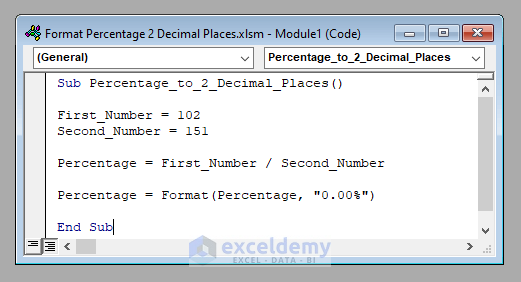# Excel VBA: Format Percentage to 2 Decimal Places

Get FREE Advanced Excel Exercises with Solutions!

In this article, I’ll show you how you can format a percentage to 2 decimal places using VBA in Excel. You’ll learn to format a percentage using VBA with examples involving a Macro and a User-Defined function (UDF).

Format Percentage to 2 Decimal Places with Excel VBA (Quick View)

``````Sub Percentage_to_2_Decimal_Places()

First_Number = 102
Second_Number = 151

Percentage = First_Number / Second_Number

Percentage = Format(Percentage, "0.00%")

End Sub``````

## How to Format Percentage to 2 Decimal Places with Excel VBA (Step-by-Step Analysis)

Without further delay, let’s go to our main discussion today. We’ll learn to format a percentage to 2 decimal places.

Let’s try to format the percentage of 102 with respect to 151 to 2 decimal places. I’m showing you the step-by-step procedure to accomplish this.

⧪ Step 1: Inserting the Inputs

First of all, we’ve to insert the inputs to calculate the percentage, Here they are 102 and 151.

``````First_Number = 102
Second_Number = 151``````

⧪ Step 2: Calculating the Percentage

Next, we’ll have to calculate the percentage. We’ll divide the first number by the second number to achieve this.

``Percentage = First_Number / Second_Number``

⧪ Step 3: Formatting to 2 Decimal Places

This is the most important step. We’ll use the Format function of VBA to convert the percentage to 2 decimal places.

``Percentage = Format(Percentage, "0.00%")``

So, the complete VBA code will be:

⧭ VBA Code:

``````Sub Percentage_to_2_Decimal_Places()

First_Number = 102
Second_Number = 151

Percentage = First_Number / Second_Number

Percentage = Format(Percentage, "0.00%")

End Sub``````⧭ Output:

Run the code with the line MsgBox Percentage (Obviously by changing the inputs according to your needs).

It’ll show the percentage of the first number with respect to the second number to 2 decimal places. Here it’s 67.55%.## Examples to Format Percentage to 2 Decimal Places with Excel VBA (Macro and UDF)

We’ve learned to percentage to 2 decimal places using VBA in Excel. Now we’ll explore a few examples to make things pretty clear.

### 1. Developing a Macro to Format a Range of Percentage to 2 Decimal Places

Here we’ve got a data set with the names of some products (B4:B13), their target sales(C4:C13), the achieved sales (D4:D13), and an empty column (E4:E13) for the percentage of the achieved sales with respect to the target sales of a company.Our objective today is to develop a Macro that’ll calculate the percentage of the achieved sales with respect to the target sales to 2 decimal places.

So, the complete VBA code will be:

⧭ VBA Code:

``````Sub Percentages_to_2_Decimal_Places()

Set First_Range = Range("D4:D13")
Set Second_Range = Range("C4:C13")
Set Output_Range = Range("E4:E13")

For i = 1 To Output_Range.Rows.Count
For j = 1 To Output_Range.Columns.Count
Output_Range.Cells(i, j) = First_Range.Cells(i, j) / Second_Range.Cells(i, j)
Output_Range.Cells(i, j) = Format(Output_Range.Cells(i, j), "0.00%")
Next j
Next i

End Sub``````⧭ Output:

Run the code (Don’t forget to change the inputs according to your need.). It’ll put the percentage of the achieved sales with respect to the target sales in the range E4:E13 of the active worksheet.### 2. Creating a User-Defined Function to Format Percentage to 2 Decimal Places with Excel VBA

We’ve learned to develop a Macro to format a range of percentages to 2 decimal places. Now we’ll create a user-defined function to accomplish this.

Therefore, the complete VBA code will be:

⧭ VBA Code:

``````Function PERCENTAGE(First_Range As Range, Second_Range As Range)

Dim Output_Range() As Variant
ReDim Output_Range(First_Range.Cells.Count - 1, First_Range.Columns.Count - 1)

For i = 1 To First_Range.Rows.Count
For j = 1 To First_Range.Columns.Count
Output_Range(i - 1, j - 1) = First_Range.Cells(i, j) / Second_Range.Cells(i, j)
Output_Range(i - 1, j - 1) = Format(Output_Range(i - 1, j - 1), "0.00%")
Next j
Next i

PERCENTAGE = Output_Range

End Function``````⧭ Output:

Select any cell of the worksheet and enter the formula:

=PERCENTAGE(D4:D13,C4:C13)It’ll calculate the percentages of the range D4:D13 with respect to the range C4:C13 and format the percentages to 2 decimal places.[It’s an Array Formula. So don’t forget to select the entire range before entering the formula and press CTRL+SHIFT+ENTER to enter it).

## Things to Remember

Here I’ve focused on formatting to 2 decimal places only. If you want to format any other decimal places, use the Format function accordingly.

For example, to format to 1 decimal place, use “0.0%” within the Format function.

Also while working with VBA in Excel, you needn’t save any file to run a VBA code. You can see the output of any code without saving the file. But in case you want to save the file for future use, you must save the file as Excel Macro-Enabled Workbook (*.xlsm).

## Conclusion

So, these are the ways to format a percentage to 2 decimal places with VBA in Excel. Do you have any questions? Feel free to ask us. And don’t forget to visit our site ExcelDemy for more posts and updates.

## Related Articles#### Rifat Hassan

Hello! Welcome to my profile. Here I will be posting articles related to Microsoft Excel. I am a passionate Electrical Engineer holding a Bachelor’s degree in Electrical and Electronic Engineering from the Bangladesh University of Engineering and Technology. Besides academic studies, I always love to keep pace with the revolution in technology that the world is rushing towards day by day. I am diligent, career-oriented, and ready to cherish knowledge throughout my life.

We will be happy to hear your thoughtsAdvanced Excel Exercises with Solutions PDF